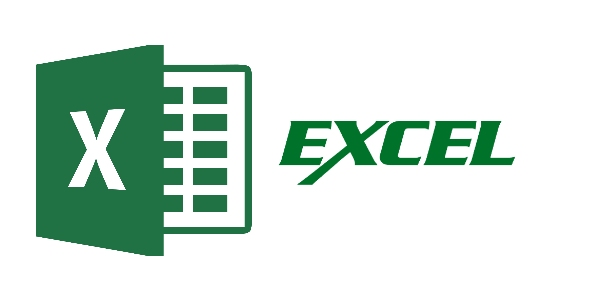# Rsa Excel Training Exam

20 Questions | Attempts: 188
ShareSettings• 1.
An easy way to sort data when there is only one sort field is to use the Sort A to Sort Z or Sort Z to A buttons
• A.

True

• B.

False

• 2.
In an IF Function the required arguments are ______________________.
• A.

Logical test and value if true, value if test is false plus formatting option

• B.

The logical test and the three possible outcomes

• C.

The Logical test plus initial outcome pus true and false

• D.

The Logical test and value if test is true, value if test is false

• 3.
You can create maximum one Excel table in a worksheet
• A.

True

• B.

False

• 4.
You cannot insert comments on each cell
• A.

True

• B.

False

• 5.
Pressing ENTER moves the selection one cell to the right by default.
• A.

True

• B.

False

• 6.
_______________ is the horizontal part of the spreadsheet.
• A.

Rows

• B.

Cell

• C.

Column

• 7.
As you begin to type a function name within a formula into a cell, a list of functions that begin with the letters you typed appears.
• A.

True

• B.

False

• 8.
You can print
• A.

An entire workbook

• B.

A single worksheet

• C.

A range of cells by range

• D.

All of the above

• 9.
What term describes explanatory text attached to a cell
• A.

Callouts

• B.

• C.

Dialogs

• D.

Extensions

• 10.
The Insert Function dialog box tells you how to use functions.
• A.

True

• B.

False

• 11.
In the following formula: =IF(A1="YES","DONE","RESTART"), what happens if A1="NO"?
• A.

Formula returns the value "DONE"

• B.

Formula will show an error

• C.

Formula returns the value "RESTART"

• D.

Formula returns the value "YES"

• 12.
Two common wildcard characters that Excel recognizes are
• A.

* and /

• B.

- and /

• C.

* and ?

• D.

+ and -

• 13.
The cell labeled F5 refers to
• A.

Row F column 5

• B.

Column F row 5

• C.

Functions available in cells

• D.

Function key F4

• 14.
The name of the active cell appears in the ____________.
• A.

Formula Bar

• B.

Name box

• C.

Column

• D.

View Tab

• 15.
When a worksheet is printed, the grid lines that surround the cells are printable by default.
• A.

True

• B.

False

• 16.
To select several cells or ranges that are not touching each other, you would _____ while selecting
• A.

Hold down the CTRL key

• B.

Hold down the SHIFT key

• C.

Hold down the ALT key

• 17.
You can select the current Row by pressing
• A.

Control + spacebar

• B.

Shift + spacebar

• C.

Alt + spacebar

• D.

Shift + Tab

• 18.
Pressing TAB moves the selection one cell to the right by default.
• A.

True

• B.

False

• 19.
The _________________ displays the name of the sheets in the workbook.
• A.

Sheet Tabs

• B.

Book Tabs

• C.

• 20.
Which of the following formulas would find the sum of the cells in column J between rows 1 and 8 in an Excel spreadsheet?
• A.

• B.

=SUM(J1:J8)

• C.

=J:1+J:8

• D.

=SUM J:1-8

## Related TopicsBack to top
×

Wait!
Here's an interesting quiz for you.